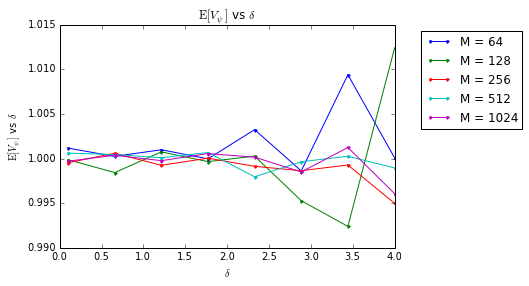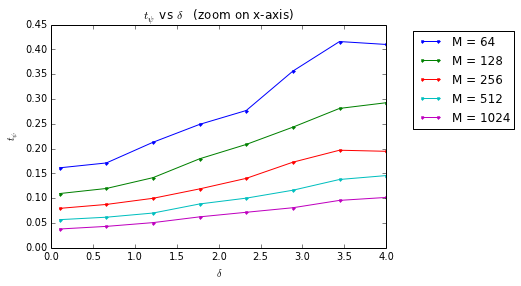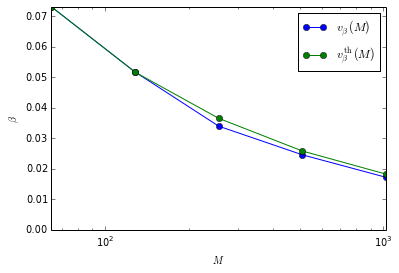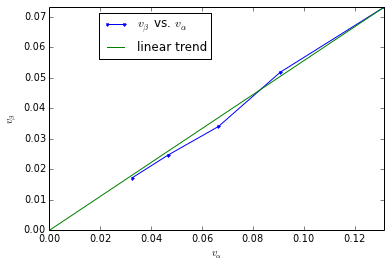# Testing a Quasi-Isometric Quantized Embedding with Percentile Analysis¶

## Introduction:¶

$$\newcommand{\bs}{\boldsymbol} \newcommand{\cl}{\mathcal} \newcommand{\bb}{\mathbb}$$

This simple python notebook aims at estimating the validity of some of the results explained in the paper

"A Quantized Johnson Lindenstrauss Lemma: The Finding of Buffon's Needle"
by Laurent Jacques (arXiv)

"In 1733, Georges-Louis Leclerc, Comte de Buffon in France, set the ground of geometric probability theory by defining an enlightening problem: What is the probability that a needle thrown randomly on a ground made of equispaced parallel strips lies on two of them? In this work, we show that the solution to this problem, and its generalization to N dimensions, allows us to discover a quantized form of the Johnson-Lindenstrauss (JL) Lemma, i.e., one that combines a linear dimensionality reduction procedure with a uniform quantization of precision $\delta>0$. In particular, given a finite set $\cl S \in \bb R^N$ of $S$ points and a distortion level $\epsilon>0$, as soon as $M > M_0 = O(\log |S|/\epsilon^2)$, we can (randomly) construct a mapping from $(\cl S, \ell_2)$ to $((\delta \bb Z)^M, \ell_1)$ that approximately preserves the pairwise distances between the points of $\cl S$. Interestingly, compared to the common JL Lemma, the mapping is quasi-isometric and we observe both an additive and a multiplicative distortions on the embedded distances. These two distortions, however, decay as $O(\sqrt{\log |S|/M})$ when $M$ increases. Moreover, for coarse quantization, i.e., for high $\delta$ compared to the set radius, the distortion is mainly additive, while for small $\delta$ we tend to a Lipschitz isometric embedding. Finally, we show that there exists "almost" a quasi-isometric embedding of $(\cl S, \ell_2)$ in $((\delta \bb Z)^M, \ell_2)$. This one involves a non-linear distortion of the $\ell_2$-distance in $\cl S$ that vanishes for distant points in this set. Noticeably, the additive distortion in this case decays slower as $O((\log S/M)^{1/4})$."

In particular, our objective is to study the behavior of a nonlinear mapping from $\bb R^N$ to $\bb R^M$, for two specific dimensions $M,N \in \bb N$. This mapping is defined as follows.

Let a random (sensing) matrix $\bs \Phi \in \bb R^{M \times N}$ be such that each component $\Phi_{ij} \sim_{\rm iid} \cl N(0,1)$, or more shorthly $\bs \Phi \sim \cl N^{M\times N}(0,1)$.

We want to combine the common random projection realized by $\bs \Phi$ with the following non-linear operation, i.e., a uniform scalar quantizer of resolution $\delta > 0$:

$$\qquad\cl Q_\delta[\lambda] = \delta \lfloor \lambda / \delta \rfloor.$$

(remark: we could define instead a midrise quantizer by adding $\delta/2$ to the definition above, and the rest of the development would be unchanged)

$$\qquad\bs \psi_\delta(\bs x) := \cl Q_\delta(\bs\Phi \bs x + \bs \xi)$$

with $\bs \xi \sim \cl U^M([0, \delta])$ is a dithering vector, i.e., a random uniform vector in $\bb R^M$ such that $u_i \sim_{\rm iid} \cl U([0, \delta])$, and $\bs \psi_\delta:\bb R^N \to (\delta \bb Z)^M$ $is our mapping of interest. In Proposition 13 (page 16) of the paper above, it shown that Proposition 13: Fix$\epsilon_0>0$,$0< \epsilon\leq \epsilon_0$and$\delta>0$. There exist two values$c,c'>0$only depending on$\epsilon_0$such that, for$\bs\Phi \sim \cl N^{M\times N}(0,1)$and$\bs \xi\sim \cl U^M([0, \delta])$, both determining the mapping$\bs\psi_\delta$above, and for$\bs u, \bs v\in\bb R^N$, $$\qquad(1 - c\epsilon) \|\bs u - \bs v\| - c'\epsilon\delta\ \leq\ \tfrac {\sqrt\pi}{\sqrt 2 M} \|\bs\psi_\delta(\bs u) - \bs\psi_\delta(\bs v)\|_1\ \leq\ (1 + c\epsilon)\|\bs u - \bs v\| + c'\epsilon\delta.\tag{1}$$ with a probability higher than$1 - 2 e^{-\epsilon^2M}$. This proposition shows that the random quantity$c_\psi M^{-1} \|\bs\psi_\delta(\bs u) - \bs\psi_\delta(\bs v)\|_1$with$c_\psi = \sqrt{\pi/2}$concentrates around its mean$\|\bs u - \bs v\|$. However, conversely to linear random projections, this concentration phenomenon displays two kind of deviation: a standard multiplicative one (the$1\pm c\epsilon$factor) and an additive one in$\pm c'\epsilon\delta$. Moreover, forcing (1) to be valid at constant probability, we deduce also that$\epsilon = O(1/\sqrt M)$, i.e., showing that both the multiplicative and the additive distortion decay as$O(1/\sqrt M)$with respect to$M$. Remark: From a standard union bound argument (see the paper), this result allows one to show the existence of a non-linear embedding from a set$\cl S \subset \bb R^N$to$(\delta \bb Z)^M$, as soon as$M \geq M_0 = O( \epsilon^{-2}\,\log |\cl S|)$. Proposition 13 is thus central to reach this property. This notebook proposes to test the behavior of the two distortions displayed in (1). For that, arbitrarily fixing$\|\bs u - \bs v\|=1$by normalizing conveniently$\bs u$and$\bs v$(e.g., after their random selection in$\bb R^N$), we study the experimental distribution of the random variable $$\qquad V_\psi = \tfrac{\sqrt\pi}{\sqrt 2 M} \|\bs\psi_\delta(\bs u) - \bs\psi_\delta(\bs v)\|_1.$$ Remark: the concentration (1) is invariant under the coordinate change$\bs u \to \lambda \bs u$,$\bs v \to \lambda \bs v$and$\delta \to |\lambda| \delta$for any$\lambda \neq 0$, which allows us to set$\|\bs u - \bs v\|=1$without any loss of generality (providing we study the variability of (1) in$\delta$). Assuming$N$fixed, we study the behavior of this random variable in function of$\delta$and$M$, i.e.,$V_\psi = V_\psi(\delta, M)$. Although$V_\psi$displays a non-trivial distribution1, we can estimate numerically the failure curve given a fixed failure probability$0 < p_{\rm fail} < 1$: $$\quad{\rm cdf}^{-1}_\psi(\delta, M;\, p_{\rm fail}) := \{t: \bb P[V_\psi(\delta, M) - 1 > t] = p_{\rm fail}\},$$ where we use the fact that$\bb E V_\psi = \|\bs u - \bs v\| = 1$We expect that, if Prop. 13 above is true, $$\qquad t_\psi(\delta,M) := {\rm cdf}^{-1}_\psi(\delta, M; p_{\rm fail}) \quad \simeq\quad a\ \epsilon(M)\ +\ b\ \epsilon(M)\ \delta,\quad \text{for some }a,b > 0,$$ and possibly find the values$a, b>0$, or their product with$\epsilon(M)$by a simple linear fit at specific values of$M$. Moreover, if$p_{\rm fail} \simeq e^{-\epsilon^2M}$holds (as in Prop. 13 above), then, once this linear fit will be obtained at several$M$s, we should also observe that$\epsilon \simeq 1/\sqrt{M}$since$p_{\rm fail}$is set to a constant value. Well, let's now test the validity of all these phenomena! 1: Actually, a mixture of *Buffon* random variable and of a Chi distribution with$N$degrees of freedom. ## The simulations:¶ Remark: launch ipython notebook with the pylab option, i.e., ipython notebook --pylab=inline In : import numpy as np from scipy import stats  Defining the$\bs \psi_\delta:\bb R^N \to (\delta \bb Z)^M$with two functions: In : def quant(val, d): # the uniform quantizer of resolution 'd' return d * np.floor(val/d)  In : def psi(vec, mat, dith, d): # the mapping (matrix A, dithering u, quantization d) return quant(mat.dot(vec) + dith, d)  The following function returns the inverse cumulative density function, i.e., given a pdf$f$associated to the random variable$X$, this function is defined as $$\quad{\rm cdf}^{-1}(p; f) = \{t: P[X > t] = \textstyle\int_{t}^{+\infty} f(u)\ {\rm d}u = p\}$$ We use the python function g(q) = stats.scoreatpercentile(samples, q)  such that $$\quad g(q) = \{t: P[X < t] = \textstyle\int_{0}^{t} f(u)\ {\rm d}u = q\}$$ where$f$is estimated experimentally from the samples. By definiton,$P[X < g(q)] = q$so that $$\quad q = P[X < g(q)] = 1 - P[X > {\rm cdf}^{-1}(p; f)] = 1 - p$$ so that, for$q = 1- p$,$g(1-p) = {\rm cdf}^{-1}(p; f)$. We can thus define this function: In : def inv_cdf(samples, p): # returns {t : P[X > t] = p} return stats.scoreatpercentile(samples, 100*(1 - p))  ### Entering into the simulations: (time for a coffee/tea break)¶ Experimental setup: The standard deviation of$V_\psi$is evaluated for several number of measurements$M$and for several values of$\delta$. For each of them a certain number of trials are generated. In the configuration, there are 10 000 trials and the sensing matrix$\bs \Phi$and the dithering$\bs \xi$are regenerated every 100 trials. In : # space dimension N = 256 # Normalising constant for the quantized embedding (see above) c_psi = np.sqrt(np.pi/2) # Number of trials nb_trials_phi = 100 # number of regeneration of Phi/dithering nb_trials_pt = 100 # number of trials for points (i.e., per phi/dithering) nb_trials = nb_trials_pt * nb_trials_phi # probability of failure for drawing the deviation curve # f(t) = {t : P[ V_psi > 1 + t ] = p_fail} p_fail = 0.05 # variable number of dimensions M_v = np.array([64, 128, 256, 512, 1024]) nb_M = M_v.size nb_delta = 8 # The fixed value for ||u - v|| = 1 dist_l2 = 1 # Testing several delta, a logspace arrangement seems logical, here from 10^-3 to 10 delta_v = np.linspace(0.1,4,nb_delta) # Curve at precentile p_fail, one per measurement V_psi_per = np.zeros([nb_M,nb_delta]) # Checking if the mean is around 1 V_psi_mean = np.zeros([nb_M,nb_delta]) # Only the standard deviation of V_psi matters here, # but interested readers could evaluate its mean too to see that it matches ||u - v||. # In this case, just uncomment this line and the one # V_psi_avg = np.zeros([nb_M,nb_delta]) for j in range(nb_M): M = M_v[j] V_psi = np.zeros(nb_trials) # temporary store distances in the embedded space for k in range(nb_delta): for i in range(nb_trials): # local variables for the loop delta = delta_v[k] # Regenerating the sensing matrix/dithering every 'nb_trials_pt' trials if (np.mod(i, nb_trials_pt) == 0): # Defining the sensing matrix and dithering Phi = np.random.randn(M,N) # and dithering, i.e., uniform random vector in [0, \delta] xi = np.random.rand(M,1)*delta # Generating two points in R^N and normalizing their distance to 1 tmp_u = np.random.randn(N,1) tmp_v = np.random.randn(N,1) tmp_dist_uv = np.linalg.norm(tmp_u - tmp_v) u = tmp_u * dist_l2 / tmp_dist_uv; v = tmp_v * dist_l2 / tmp_dist_uv; # projecting the two points according to psi u_proj = psi(u, Phi, xi, delta); v_proj = psi(v, Phi, xi, delta); # storing one sample of the normalized l1 distance V_psi[i] = c_psi*np.linalg.norm(u_proj - v_proj,1)/M # Finding the percentile curve V_psi_per[j,k] = inv_cdf(V_psi - 1, p_fail) # For mean evaluation, you could estimate too: V_psi_mean[j,k] = np.mean(V_psi) print j, # observing the loop progression (sober progress bar ;-)   0 1 2 3 4  Let's observe now the diferent evolutions of$V_{\psi}$vs.$\delta$for the different number of measurements$M\in \{64, \cdots, 1024\}$selected above: In : # Mean plot fig, ax = plt.subplots() for k in range(M_v.size): ax.plot(delta_v, V_psi_mean[k], '.-', label = "M = %i" % M_v[k]) ax.set_title(r'${\rm E}[V_\psi]$vs$\delta$') ax.set_xlabel(r'$\delta$') ax.set_ylabel(r'${\rm E}[V_\psi]$vs$\delta$') ax.legend(bbox_to_anchor=(1.40, 1)) # Percentile plot fig, ax = plt.subplots() for k in range(M_v.size): ax.plot(delta_v, V_psi_per[k], '.-', label = "M = %i" % M_v[k]) ax.set_title(r'$t_\psi$vs$\delta$(zoom on x-axis)') ax.set_xlabel(r'$\delta$') ax.set_ylabel(r'$t_\psi$') ax.legend(bbox_to_anchor=(1.40, 1))  Out: <matplotlib.legend.Legend at 0x10ebcd990>From this last plot, we conclude that: • for$\delta \gtrsim 0.2$,$t_\psi(\delta,M)$displays roughly a linear progression in$\delta$; • for different value of$M$, this linear trend has an offset as$\delta \simeq 0$; • the slope and the offset of the linear progresison clearly depend on$M$. Let's go one step further in our analysis. From this plot, we perform a linear fit to estimate$t_\psi(\delta,M)$as $$\quad t_\psi(\delta,M)\quad \simeq\quad v_\alpha +\ v_\beta\ \delta,\qquad \text{for some }v_\alpha, v_\beta > 0.$$ Actually, we have to estimate one couple of$(v_\alpha,v_\beta)$for each value$M$, since, from what we explained above, we should have $$\qquad v_\alpha\ =\ a\ \epsilon$$ and $$\qquad v_\beta\ =\ b\ \epsilon$$ for some constants$a$and$b$and$\epsilon = O(1/\sqrt{M})$depending on$M$(at fixed failure probability for inequality (1) above). Let us see if this is what we get. We first do the linear fit: In : # initialization v_alpha = np.zeros(M_v.size) v_beta = np.zeros(M_v.size) # For each M, use np.polyfit at degree 1 for k in range(M_v.size): v_beta[k], v_alpha[k] = np.polyfit(delta_v, V_psi_per[k], 1)  If$v_\beta = b \epsilon$, where we recall that$v_\beta$is the slope of each curves in the plots above, we should see a good match between$v_\beta(M)$and $$\qquad v^{\rm th}_\beta(M)\ :=\ \sqrt{\tfrac{M_0}{M}}\ v_\beta(M_0),\qquad \text{with v^{\rm th}_\beta(M_0)=v_\beta(M_0)}$$ for one fixed value$M_0$. Is it what we have? In : plt.semilogx(M_v, v_beta, 'o-', label=r'$v_\beta(M)$') plt.semilogx(M_v, v_beta*np.sqrt(M_v)/np.sqrt(M_v), 'o-', label=r'$v^{\rm th}_\beta(M)$') # M_0 is the first M of M_v plt.xlabel(r'$M$') plt.ylabel(r'$\beta$') plt.axis('tight') plt.ylim(0, v_beta) plt.legend();The match sounds good enough! Finally, if we really have$v_\alpha = a \epsilon$and$v_\beta = b \epsilon$,$v_\beta$should be a linear function of$v_\alpha$, i.e.,$v_\alpha/v_\beta \simeq a/b$. Let's test this fact. In : fig, ax = plt.subplots() ax.plot(v_alpha, v_beta, '.-', label = r'$v_\beta$vs.$v_\alpha$') ax.plot(np.linspace(0,v_alpha.max(),10), np.linspace(0,v_alpha.max(),10)*v_beta.max()/v_alpha.max(), label = 'linear trend') ax.set_xlim(0, v_alpha.max()) ax.set_ylim(0, v_beta.max()) ax.set_xlabel(r'$v_\alpha$') ax.set_ylabel(r'$v_\beta\$')
ax.legend(bbox_to_anchor=(.5, 1))

ratio, offset = np.polyfit(v_alpha,v_beta,1)
print "We approximatley have v_beta = %f v_alpha + %f" % (ratio, offset)

We approximatley have v_beta = 0.574426 v_alpha + -0.002124Laurent Jacques, November 2013